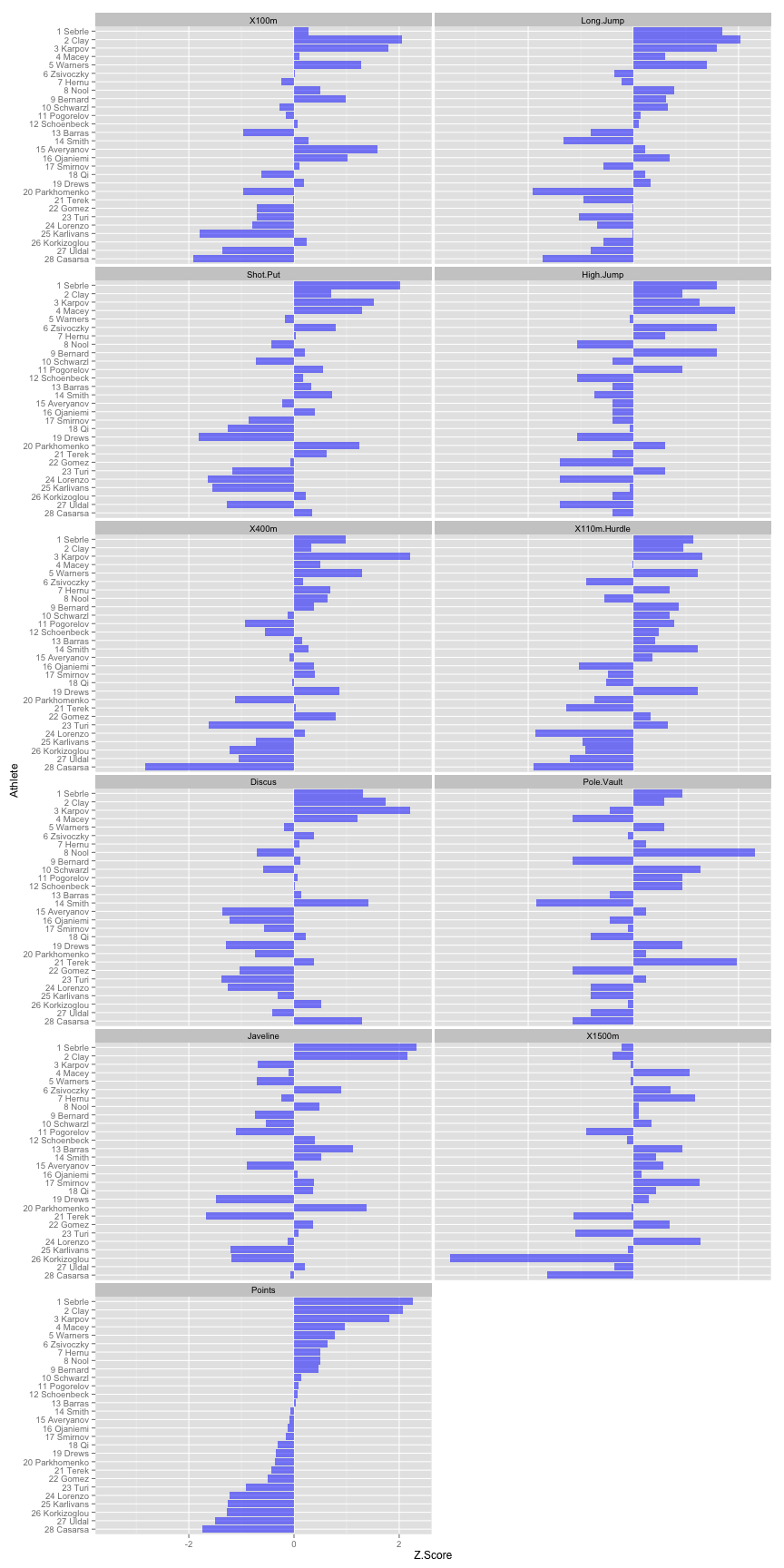Playbook: Exploring decathlon competition data [Part 1]

# Playbook: Exploring Decathlon Competition Data [Part 1]

## Data set

Decathlon data set comes from FactoMineR package and represents two competitions: Decastar and Olympic Games. For this example we will explore only Olympic Games competition, so we need to subset the data.

``````# Load the needed packages
library(FactoMineR)
library(ggplot2)
library(reshape2)

data(decathlon)

# Subset the data so it only includes Olympic Games
decathlon <- subset(decathlon, Competition == "OlympicG")

# Add the Rank to the athlete in the row names
rownames(decathlon) <- paste(1:28, rownames(decathlon))

# Fix the column (events) names so they don't start with a number
names(decathlon) <- c("X100m", "Long.Jump", "Shot.Put", "High.Jump", "X400m",
"X110m.Hurdle", "Discus", "Pole.Vault", "Javeline", "X1500m", "Rank", "Points",
"Competition")
``````

Using googleVis package we can create interactive table

``````# Create copy of decathlon data, but add column Athlete and it's Rank
decathlon.with.athlete.name <- data.frame(Athlete = factor(row.names(decathlon),
levels = row.names(decathlon)), decathlon)

# Remove the Rank column and Competition column
decathlon.with.athlete.name\$Rank <- NULL
decathlon.with.athlete.name\$Competition <- NULL

# Create the interactive table
dec.table <- gvisTable(decathlon.with.athlete.name, options = list(height = 500,
width = 800))

print(dec.table, "chart")
``````

## Exploring the data

Before we start modeling the data it is always a good thing to visualize it. Before we do that, we need to melt the data, from wide format to long, so the ggplot2 can use it

``````# Conver the data from wide to long format
decathlon.long <- melt(decathlon.with.athlete.name, id.vars = "Athlete", value.name = "Score",
variable.name = "Event")

# Plot the data using ggplot2
gg <- ggplot(decathlon.long, aes(y = Score, x = 1))
gg <- gg + geom_violin(alpha = 0.2, fill = "blue")
gg <- gg + facet_wrap(~Event, ncol = 3, scales = "free_y")
gg <- gg + geom_point(alpha = 0.7)
gg
``````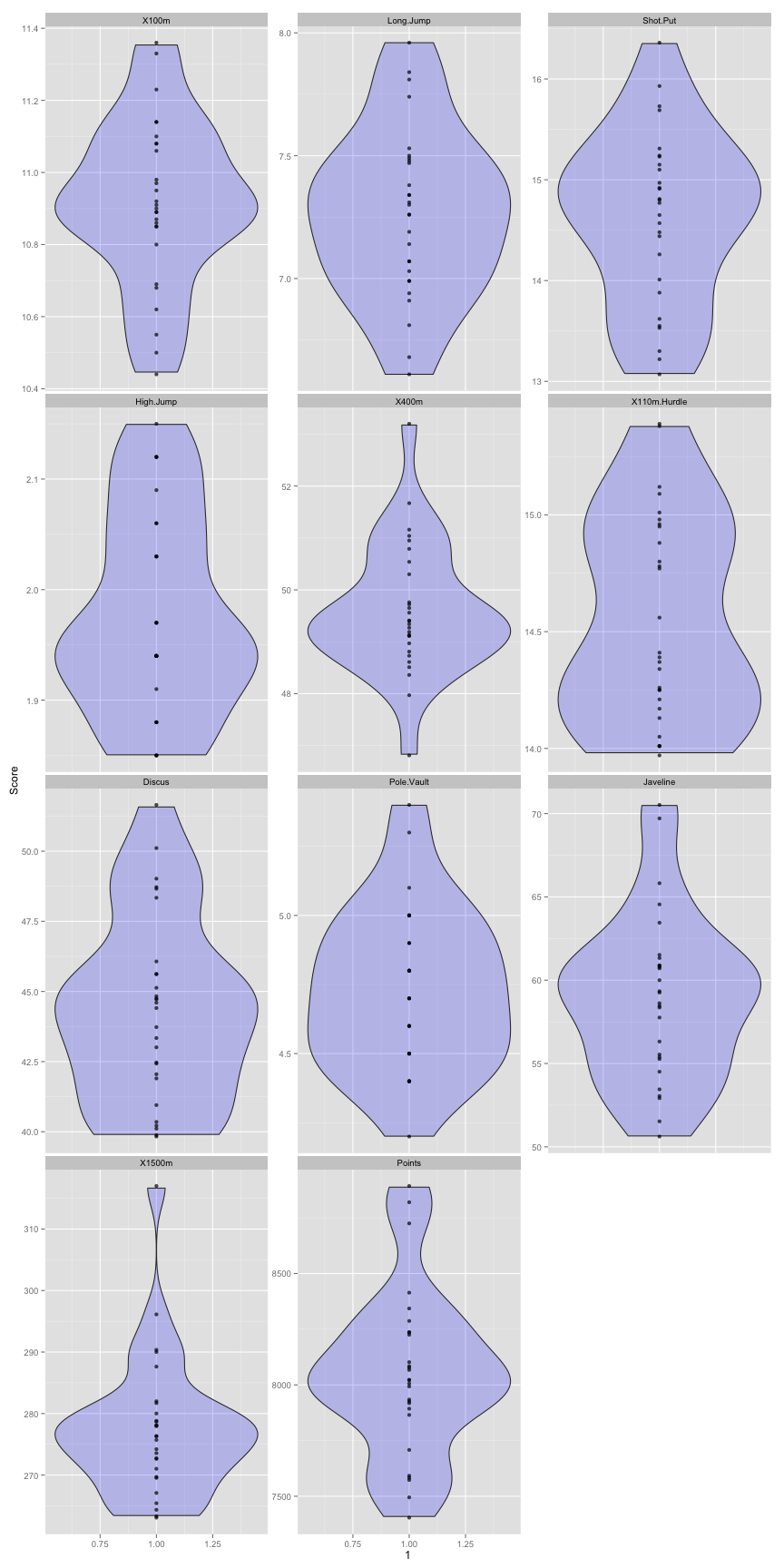## Multiple regression: what event influence the total score the most?

From the given data we are interested in creating a model that predicts the total score from event scores.

``````# Create regression model to predict Points from 10 events
model1 <- lm(Points ~ X100m + Long.Jump + Shot.Put + High.Jump + X400m + X110m.Hurdle +
Discus + Pole.Vault + Javeline + X1500m, decathlon)

summary(model1)
``````
``````##
## Call:
## lm(formula = Points ~ X100m + Long.Jump + Shot.Put + High.Jump +
##     X400m + X110m.Hurdle + Discus + Pole.Vault + Javeline + X1500m,
##     data = decathlon)
##
## Residuals:
##    Min     1Q Median     3Q    Max
## -6.296 -1.240 -0.217  1.367  5.373
##
## Coefficients:
##              Estimate Std. Error t value Pr(>|t|)
## (Intercept)  8639.559     76.074   113.6   <2e-16 ***
## X100m        -227.378      4.734   -48.0   <2e-16 ***
## Long.Jump     243.110      3.494    69.6   <2e-16 ***
## Shot.Put       61.534      1.369    45.0   <2e-16 ***
## High.Jump     897.790     10.411    86.2   <2e-16 ***
## X400m         -47.192      1.102   -42.8   <2e-16 ***
## X110m.Hurdle -121.144      1.854   -65.3   <2e-16 ***
## Discus         21.153      0.307    68.8   <2e-16 ***
## Pole.Vault    295.458      2.634   112.2   <2e-16 ***
## Javeline       15.094      0.164    92.0   <2e-16 ***
## X1500m         -6.014      0.099   -60.8   <2e-16 ***
## ---
## Signif. codes:  0 '***' 0.001 '**' 0.01 '*' 0.05 '.' 0.1 ' ' 1
##
## Residual standard error: 3.33 on 17 degrees of freedom
## Multiple R-squared:     1,   Adjusted R-squared:     1
## F-statistic: 3.38e+04 on 10 and 17 DF,  p-value: <2e-16
``````

As can be seen from the R squared (variance explained) the model explains 99.9% of the variance in the data and all coeficients are statistically significant (p<0.001).

What we are interested next is what event influences the total points the most?

To do that we need to convert our coeficients to beta coeficients. Beta coeficients represent standardize coeficients (same as we have entered that data in Z-scores, which we will to compare) and as such we can compare between different events.

``````# Load package with the function to convert coeficients to beta
suppressPackageStartupMessages(library(QuantPsyc))

model1.beta.coeficients <- lm.beta(model1)

round(model1.beta.coeficients, 2)
``````
``````##        X100m    Long.Jump     Shot.Put    High.Jump        X400m
##        -0.14         0.22         0.14         0.22        -0.16
## X110m.Hurdle       Discus   Pole.Vault     Javeline       X1500m
##        -0.14         0.19         0.23         0.20        -0.18
``````

As we can see from the beta coeficients, the most influence on the total score has the Pole Vault (0.23), which means that if we improve Pole Vault for one SD (which is 0.29 meters) we increase total score by 0.23 SD (which is 85.69 points)

For the sake of an example, let’s create the same model, but this time normalize the scores beforehand

``````# Normalize the decatlhon data (except first colum which is name)
decathlon.normal <- as.data.frame(scale(decathlon.with.athlete.name[-1]))

decathlon.normal <- data.frame(Athlete = factor(row.names(decathlon), levels = row.names(decathlon)),
decathlon.normal)

# Create regression model using normalized scores
model2 <- lm(Points ~ X100m + Long.Jump + Shot.Put + High.Jump + X400m + X110m.Hurdle +
Discus + Pole.Vault + Javeline + X1500m, decathlon.normal)

# Get the coeficients
round(coef(model2), 2)
``````
``````##  (Intercept)        X100m    Long.Jump     Shot.Put    High.Jump
##         0.00        -0.14         0.22         0.14         0.22
##        X400m X110m.Hurdle       Discus   Pole.Vault     Javeline
##        -0.16        -0.14         0.19         0.23         0.20
##       X1500m
##        -0.18
``````

As can be seen above, the coeficients with normalized data and beta coeficients are the same. Hence, the beta coeficients represent normalized coeficients that can be used to compare strength of each coeficient as we did before.

You have probably noticed that all distance events have negative coeficient. That’s because they represent less is better type of score. The lower your time in 100m run the better.

We need to convert those so we can compare events and create athlete profilese where higher Z-score means better results.

To do that we juts need to multiply normalized distance events scores by -1

``````decathlon.normal[, c("X100m", "X400m", "X110m.Hurdle", "X1500m")] <- decathlon.normal[,
c("X100m", "X400m", "X110m.Hurdle", "X1500m")] * -1
``````

We can proceed by making a regression model again

``````model3 <- lm(Points ~ X100m + Long.Jump + Shot.Put + High.Jump + X400m + X110m.Hurdle +
Discus + Pole.Vault + Javeline + X1500m, decathlon.normal)

summary(model3)
``````
``````##
## Call:
## lm(formula = Points ~ X100m + Long.Jump + Shot.Put + High.Jump +
##     X400m + X110m.Hurdle + Discus + Pole.Vault + Javeline + X1500m,
##     data = decathlon.normal)
##
## Residuals:
##       Min        1Q    Median        3Q       Max
## -0.016897 -0.003329 -0.000583  0.003670  0.014420
##
## Coefficients:
##               Estimate Std. Error t value Pr(>|t|)
## (Intercept)  -3.03e-17   1.69e-03     0.0        1
## X100m         1.41e-01   2.94e-03    48.0   <2e-16 ***
## Long.Jump     2.23e-01   3.20e-03    69.6   <2e-16 ***
## Shot.Put      1.41e-01   3.14e-03    45.0   <2e-16 ***
## High.Jump     2.17e-01   2.51e-03    86.2   <2e-16 ***
## X400m         1.61e-01   3.75e-03    42.8   <2e-16 ***
## X110m.Hurdle  1.44e-01   2.20e-03    65.3   <2e-16 ***
## Discus        1.87e-01   2.72e-03    68.8   <2e-16 ***
## Pole.Vault    2.29e-01   2.05e-03   112.2   <2e-16 ***
## Javeline      2.02e-01   2.19e-03    92.0   <2e-16 ***
## X1500m        1.83e-01   3.01e-03    60.8   <2e-16 ***
## ---
## Signif. codes:  0 '***' 0.001 '**' 0.01 '*' 0.05 '.' 0.1 ' ' 1
##
## Residual standard error: 0.00894 on 17 degrees of freedom
## Multiple R-squared:     1,   Adjusted R-squared:     1
## F-statistic: 3.38e+04 on 10 and 17 DF,  p-value: <2e-16
``````

As can be seen the coeficients between model2 and model3 are the same, only the distance coeficeints are multiplied by -1 (hence they are positive)

``````model.coeficients <- data.frame(model2 = coef(model2), model3 = coef(model3))
round(model.coeficients, 2)
``````
``````##              model2 model3
## (Intercept)    0.00   0.00
## X100m         -0.14   0.14
## Long.Jump      0.22   0.22
## Shot.Put       0.14   0.14
## High.Jump      0.22   0.22
## X400m         -0.16   0.16
## X110m.Hurdle  -0.14   0.14
## Discus         0.19   0.19
## Pole.Vault     0.23   0.23
## Javeline       0.20   0.20
## X1500m        -0.18   0.18
``````

Let’s plot the strength of each event (coeficient) influencing the total score

``````model.coeficients <- data.frame(Event = rownames(model.coeficients), beta = coef(model3))

# Sort the Event factor based on value
model.coeficients\$Event <- with(model.coeficients, factor(Event, levels = Event[order(beta)]))

gg <- ggplot(model.coeficients, aes(x = Event, y = beta))
gg <- gg + geom_bar(stat = "identity", fill = "blue", alpha = 0.5)
gg <- gg + coord_flip()
gg
``````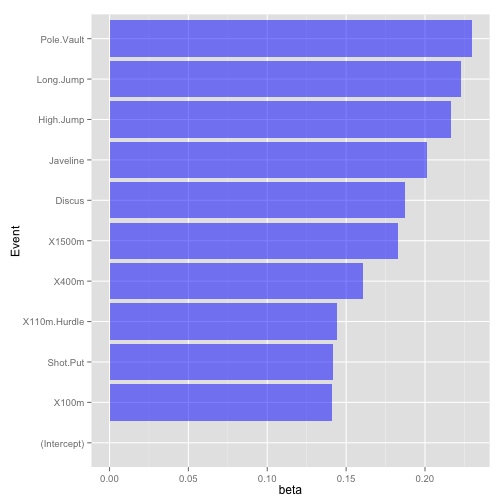## What event to train/improve to reap the most points?

The next thing to do and to make it more usable to coaches is to calculate the smallest worthwile change (SWC) of the events. To do that we need to calculate SWC of the total score, where SWC = 0.2 x SD

``````SWC <- sd(decathlon\$Points) * 0.2
``````

Using SWC of the total points (74.51 points), we calculate change in each event that will result in that change

``````# Divide SWC in total point by each coeficient (except intercept)
event.SWC <- SWC/coef(model1)[-1]

event.SWC <- data.frame(Event = names(event.SWC), SWC = event.SWC)
rownames(event.SWC) <- NULL

event.SWC
``````
``````##           Event      SWC
## 1         X100m  -0.3277
## 2     Long.Jump   0.3065
## 3      Shot.Put   1.2109
## 4     High.Jump   0.0830
## 5         X400m  -1.5790
## 6  X110m.Hurdle  -0.6151
## 7        Discus   3.5226
## 8    Pole.Vault   0.2522
## 9      Javeline   4.9369
## 10       X1500m -12.3911
``````

Using this table coaches estimate what improvement in each event can yield SWC in total score. Together with the knowledge of ‘adaptability’ of the athlete in each event (how much can he improve), the knowlede of typical variability (typical error) of the event (for each athlete from competition to competition), using this table can help coaches in decision making (i.e. what event to emphasize in training).

For example, is it easier to strip 0.33 seconds of 100m run or to gain 3.52m in the discuss to increase total score by worthwhile amount?

One way to show how hard this might be woud be to compare SWC for each event individually (SD x 0.2) and above calculated SWCs for total scores.

``````event.SWC\$Event.SWC <- as.numeric(sapply(decathlon.with.athlete.name[2:11],
sd) * 0.2)

# Calculate the hardness score
event.SWC <- within(event.SWC, hardness <- abs(SWC/Event.SWC))

event.SWC
``````
``````##           Event      SWC Event.SWC hardness
## 1         X100m  -0.3277   0.04621    7.093
## 2     Long.Jump   0.3065   0.06823    4.492
## 3      Shot.Put   1.2109   0.17124    7.071
## 4     High.Jump   0.0830   0.01799    4.614
## 5         X400m  -1.5790   0.25369    6.224
## 6  X110m.Hurdle  -0.6151   0.08854    6.947
## 7        Discus   3.5226   0.65992    5.338
## 8    Pole.Vault   0.2522   0.05788    4.358
## 9      Javeline   4.9369   0.99517    4.961
## 10       X1500m -12.3911   2.26437    5.472
``````

What hardness score is telling us is how many SWCs each event needs to improve to yield SWC in the total score.

Let’s take Discuss throw as an example: to improve total score for SWC (74.51 points), one needs to increase discuss distance for 3.52m. The SWC (between individual SD for discss ) for discuss event solely is 0.66m. Hence one needs 3.52 / 0.66 = 5.33 discuss smallest worthwhile improvements to yield SWC in the total score.

Let’s create the graph

``````event.SWC\$Event <- with(event.SWC, factor(Event, levels = Event[order(hardness,
decreasing = TRUE)]))

gg <- ggplot(event.SWC, aes(x = Event, y = hardness))
gg <- gg + geom_bar(stat = "identity", fill = "blue", alpha = 0.5)
gg <- gg + coord_flip()
gg
``````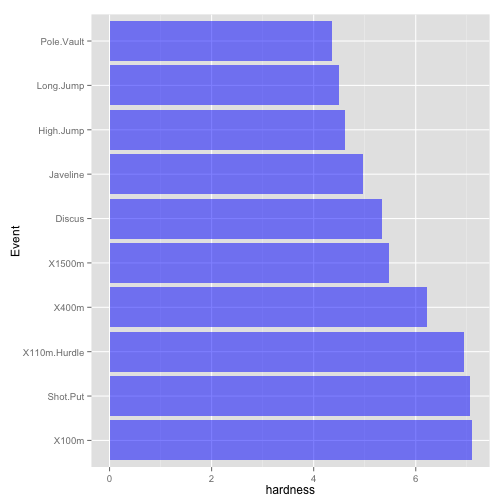And you have guessed correctly – this graph is same as beta coeficients (double check above). Thus one can use beta coeficients to understand how hard migh be to change each evet to get SWC in the total score

## Athlete Profiles

Using the normalized event scores we can draw profile for each athlete and see his strengths and weaknesses compared to other competitiors.

``````# Convert normalized data from wide to long format needed for ggplot2
decathlon.normal.long <- melt(decathlon.normal, id.vars = "Athlete", value.name = "Z.Score",
variable.name = "Event")

gg <- ggplot(decathlon.normal.long, aes(x = Event, y = Z.Score))
gg <- gg + geom_bar(stat = "identity", fill = "blue", alpha = 0.5)
gg <- gg + coord_flip()
gg <- gg + facet_wrap(~Athlete, ncol = 3)
gg
``````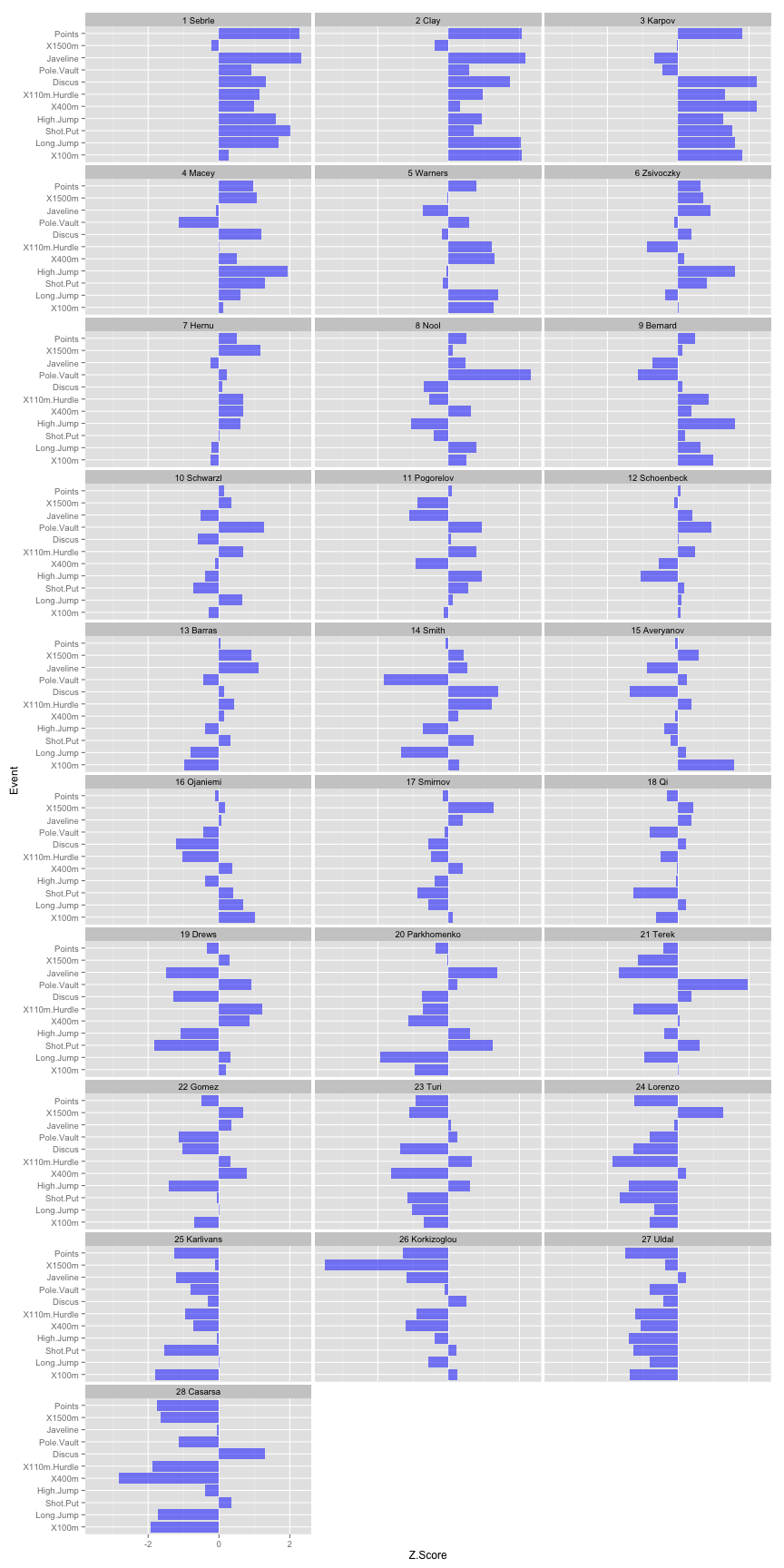And we can do the same for each event, so we can compare the athletes for each.

``````# Reverse the order of the athlete so the graph is sorted from the best to
# the worst
decathlon.normal.long\$Athlete = with(decathlon.normal.long, factor(Athlete,
levels = rev(levels(Athlete))))

gg <- ggplot(decathlon.normal.long, aes(x = Athlete, y = Z.Score))
gg <- gg + geom_bar(stat = "identity", fill = "blue", alpha = 0.5)
gg <- gg + coord_flip()
gg <- gg + facet_wrap(~Event, ncol = 2)

# Reverse the order of the factors
decathlon.normal.long\$Athlete = with(decathlon.normal.long, factor(Athlete,
levels = rev(levels(Athlete))))

# Plot
gg
``````